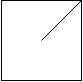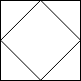# LeetCode Regions Cut By Slashes Solution

An `n x n` grid is composed of `1 x 1` squares where each `1 x 1` square consists of a `'/'`, `'\'`, or blank space `' '`. These characters divide the square into contiguous regions.

Given the grid `grid` represented as a string array, return the number of regions.

Note that backslash characters are escaped, so a `'\'` is represented as `'\\'`.

Example 1:Input: `grid = [" /","/ "]`
Output: `2`
Example 2:Input: `grid = [" /"," "]`
Output: `1`
Example 3:Input: `grid = ["/\\\\","\\\\/"]`
Output: `5`
Explanation: Recall that because \ characters are escaped, "\\/" refers to \/, and "/\\" refers to /\.

Constraints:

• `n == grid.length == grid[i].length`
• `1 <= n <= 30`
• `grid[i][j]` is either `'/'`, `'\'`, or `' '`.

## Solution

With the cutted sections, we will join the sections depending on the slashes. For example, '/' means that `north` joins `west` and `south` joins `east`. Note that we must also join the `north` region of a square to the `south` region of the square to the top of it. Similar for the left square's `east` region and current square's `west` region.

This is best approached by DSU, in the very end after joining all sections, we just have to find the number of components in the map `regions`.

#### Implementation

``````1def regionsBySlashes(grid: List[str]) -> int:
2    regions = {}
3    def find(x):
4        y = regions.get(x, x)
5        if y != x:
6            regions[x] = y = find(y)
7        return y
8    def union(x, y):
9        regions[find(x)] = find(y)
10
11    size = len(grid)
12    for i in range(size):
13        for j in range(size):
14            if i > 0:           # connect square with top
15                union((i - 1, j, 'S'), (i, j, 'N'))
16            if j > 0:           # connect square with left
17                union((i, j - 1, 'E'), (i, j, 'W'))
18            if grid[i][j] != "/":             # ' ' or '\'
19                union((i, j, 'N'), (i, j, 'E'))   # connect NE
20                union((i, j, 'S'), (i, j, 'W'))   # connect SW
21            if grid[i][j] != "\\":            # ' ' or '/'
22                union((i, j, 'N'), (i, j, 'W'))   # connect NW
23                union((i, j, 'S'), (i, j, 'E'))   # connect SE
24    return len(set(map(find, regions)))``````

Alternatively, we can find the number of connected components as in Number of Coneected Components, but bare in mind that we should only decrease the component count when we know that two elements aren't from the same set.